#### Thank you for registering.

One of our academic counsellors will contact you within 1 working day.

Please check your email for login details.

Click to Chat

1800-1023-196

+91-120-4616500

CART 0

• 0
MY CART (5)

Use Coupon: CART20 and get 20% off on all online Study Material

ITEM
DETAILS
MRP
DISCOUNT
FINAL PRICE
Total Price: Rs.

There are no items in this cart.
Continue Shopping• Complete JEE Main/Advanced Course and Test Series
• Offered Price: Rs. 15,900
• View Details

# Chapter 4: Triangles Exercise – 4.6

### Question: 1

Triangles ABC and DEF are similar.

(i) If area of (ΔABC) = 16 cm2, area (ΔDEF) = 25 cmand BC = 2.3 cm, find EF.

(ii) If area (ΔABC) = 9 cm2, area (ΔDEF) = 64 cmand DE = 5.1 cm, find AB.

(iii) If AC = 19 cm and DF = 8 cm, find the ratio of the area of two triangles.

(iv) If area of (ΔABC) = 36 cm2, area (ΔDEF) = 64 cmand DE = 6.2 cm, find AB.

(v) If AB = 1.2 cm and DE = 1.4 cm, find the ratio of the area of two triangles.

### Solution:

(i) We know that the ratio of areas of two similar triangles is equal to the ratio of squares of their corresponding sides.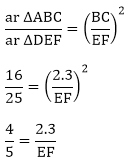EF = 2.875 cm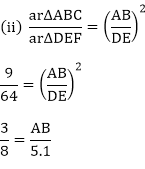AB = 1.9125 cm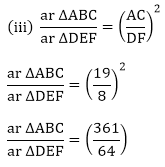AB = 4.65 cm### Question: 2

In the fig 4.178, ΔACB is similar to ΔAPQ. If BC = 10 cm, PQ = 5 cm, BA = 6.5 cm, AP = 2.8 cm, find CA and AQ. Also, find the Area of ΔACB: Area of ΔAPQ.

### Solution:

Given:  ΔACB is similar to ΔAPQ

BC = 10 cm

PQ = 5 cm

BA = 6.5 cm

AP = 2.8 cm

Find:

(1) CA and AQ

(2) Area of Δ ACB: Area of ΔAPQ

(1) It is given that Δ ACB – ΔAPQ

We know that for any two similar triangles the sides are proportional. Hence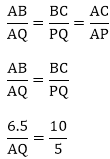AQ = 3.25 cm

Similarly,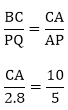CA = 5.6 cm

(2) We know that the ratio of areas of two similar triangles is equal to the ratio of squares of their corresponding sides.### Question: 3

The areas of two similar triangles are 81 cm² and 49 cm² respectively. Find the ration of their corresponding heights. What is the ratio of their corresponding medians?

### Solution:

Given: The area of two similar triangles is 81cm2 and 49cm2 respectively.

To find:

(1) The ratio of their corresponding heights.

(2) The ratio of their corresponding medians.

(1) We know that the ratio of areas of two similar triangles is equal to the ratio of squares of their corresponding altitudes.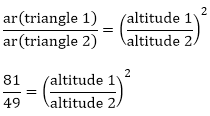Taking square root on both sides, we get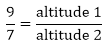Altitude 1: altitude 2 = 9: 7

(2) We know that the ratio of areas of two similar triangles is equal to the ratio of squares of their medians.Taking square root on both sides, we getMedian 1: median 2 = 9: 7

### Question: 4

The areas of two similar triangles are 169 cmand 121 cmrespectively. If the longest side of the larger triangle is 26 cm, find the longest side of the smaller triangle.

### Solution:

Given:

The area of two similar triangles is 169 cm2 and 121 cm2 respectively. The longest side of the larger triangle is 26 cm.

To find:

Longest side of the smaller triangle

We know that the ratio of areas of two similar triangles is equal to the ratio of squares of their corresponding sides.Taking square root on both sides, we get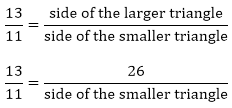Side of the smaller triangleHence, the longest side of the smaller triangle is 22 cm.

### Question: 5

The corresponding altitudes of two similar triangles are 6 cm and 9 cm respectively. Find the ratio of their areas.

### Solution:

Given:

The area of two similar triangles is 25 cm2 and 36 cm2 respectively. If the altitude of first triangle 2.4 cm.

To find:

The altitude of the other triangle

We know that the ratio of areas of two similar triangles is equal to the ratio of squares of their corresponding altitudes.Taking square root on both sides, we getAltitude 2 = 2.88 cm

Hence, the corresponding altitude of the other is 2.88 cm.

### Question: 6

ABC is a triangle in which ∠A = 90°, AN⊥BC, BC = 12 cm and AC = 5 cm. Find the ratio of the areas of ΔANC and ΔABC.

### Solution:

Given:

The corresponding altitudes of two similar triangles are 6 cm and 9 cm respectively.

To find:

Ratio of areas of triangle

We know that the ratio of areas of two similar triangles is equal to the ratio of squares of their corresponding altitudes.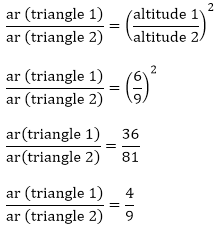ar (triangle 1): ar (triangle 2)

= 4 : 9

Hence, the ratio of the areas of two triangles is 4: 9.

### Question: 7

ABC is a triangle in which ∠A°, AN ⊥ BC, BC = 12 cm and AC = 5 cm. Find the ratio of the areas of ΔANC and ΔABC.

### Solution:

Given:

In ΔABC, ∠A = 90°, AN⊥BC, BC = 12 cm and AC = 5 cm.

To find:
Ratio of the triangles ΔANC and ΔABC.

In ΔANC and ΔABC,

∠ACN = ∠ACB (Common)

∠A = ∠ANC (90°90°)

Therefore, ΔANC –  ΔABC (A similarity)

We know that the ratio of areas of two similar triangles is equal to the ratio of squares of their corresponding sides.

Therefore,### Question: 8

In Fig, DE || BC

(i) If DE = 4 m, BC = 6 cm and Area (Δ ADE) = 16 cm2 (Δ ADE) = 16 cm2, find the area of ΔABC.

(ii) If DE = 4 cm, BC = 8 cm and Area (Δ ADE) = 25 cm2 (Δ ADE) = 25 cm2, find the area of ΔABC.

(iii) If DE: BC = 3: 5

Calculate the ratio of the areas of Δ ADE and the trapezium BCED.

### Solution:

In the given figure, we have DE ∥ BC.

In ΔADE and ΔABC ΔADE and ΔABC

∠ADE = ∠B (Corresponding angles)

∠DAE = ∠BAC (Common)

So, ΔADE and ΔABC (A Similarity)

(i) We know that the ratio of areas of two similar triangles is equal to the ratio of squares of their corresponding sides.

Hence,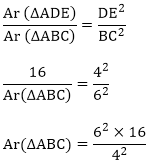Ar (ΔABC) = 36 cm2

(ii) We know that the ratio of areas of two similar triangles is equal to the ratio of squares of their corresponding sides.

Hence,(iii) We know thatLet the area of ΔADE = 9x sq units

Area of ΔABC = 25 x sq units Now,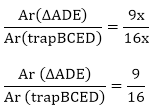### Question: 9

In ΔABC, D and E are the mid- points of AB and AC respectively. Find the ratio of the areas ΔADE and ΔABC.

### Solution:

Given:

In ΔABC, D and E are the midpoints of AB and AC respectively.

To find:

Ratio of the areas of ΔADE and ΔABC

It is given that D and E are the midpoints of AB and AC respectively.

Therefore, DE II BC (Converse of mid-point theorem)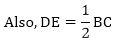In ΔADE and ΔABC

∠ADE = ∠B(Corresponding angles)

∠DAE = ∠BAC (common)

So, ΔADE – ΔABC (AA Similarity)

We know that the ratio of areas of two similar triangles is equal to the ratio of square of their corresponding sides.### Question: 10

The areas of two similar triangles are 100 cm2 and 49 cm2 respectively. If the altitude of the bigger triangles is 5 cm, find the corresponding altitude of the other.

### Solution:

Given: the area of the two similar triangles is 100 cm2 and 49cm2 respectively. If the altitude of the bigger triangle is 5 cm

To find: their corresponding altitude of the other triangle

We know that the ratio of the areas of the two similar triangles is equal to the ratio of squares of their corresponding altitudes.Taking squares on both the sides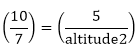Altitude 2 = 3.5 cm

### Question: 11

The areas of two similar triangles are 121 cm2 and 64 cm2 respectively. If the median of the first triangle is 12.1 cm, find the corresponding median of the other.

### Solution:

Given: the area of the two triangles is 121cm2 and 64cm2 respectively. If the median of the first triangle is 12.1cm

To find the corresponding medians of the other triangle

We know that ratio of the areas of the two similar triangles are equal to the ratio of the squares of their mediansTaking the squareroot on the both sides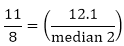Median 2 = 8.8 cm

### Question: 12

If ΔABC ∼ ΔDEF such that AB = 5cm, area (ΔABC) = 20 cm2 and area (ΔDEF) = 45 cm2, determine DE.

### Solution:

Given: the area of the two similar ΔABC = 20cm2 and ΔDEF = 45cm2 and AB = 5cm

To measure of DE

We know that the ratio of the areas of the two similar triangles is equal to the ratio of the squares of their corresponding sides.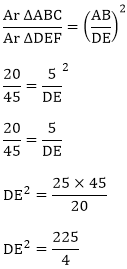DE = 7.5 cm

### Question: 13

In ΔABC, PQ is a line segment intersecting AB at P and AC at Q such that PQ ∥ BC and PQ divides ΔABC into two parts equal in area. Find BP/AB.

### Solution:

Given: in ΔABC, PQ is a line segment intersecting AB at P and AC at such that PQ ∥ BC and PQ divides ΔABC in two parts equal in area

To find: BP/AB

We have PQ ∥ BC and

Ar(ΔAPQ) = Ar(quad BPQC)

Ar(ΔAPQ) + Ar(ΔAPQ) = Ar(quad BPQC) + Ar(ΔAPQ)

2(Ar (ΔAPQ) = Ar(ΔABC)

Now PQ ∥ BC and BA is a transversal

In ΔABC and ΔAPQ)

∠APQ = ∠B (corresponding angles)

∠PAQ = ∠BAC (common)

In ΔABC ∼ ΔAPQ) (A similarity)

We know that the ratio of the areas of the two similar triangles is used and is equal to the ratio of their squares of the corresponding sides.

Hence### Question: 14

The areas of two similar triangles ABC and PQR are in the ratio 9: 16. If BC = 4.5 cm, find the length of QR.

### Solution:

Given: the areas of the two similar triangles ABC and PQR are in the ratio 9:16. BC = 4.5 cm

To find: Length of QR

We know that the ratio of the areas of the two similar triangles is equal to the ratio of the squares of their corresponding sides.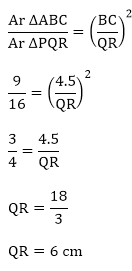### Question: 15

ABC is a triangle and PQ is a straight line meeting AB and P and AC in Q. If AP = 1 cm, PB = 3 cm, AQ = 1.5 cm, QC = 4.5 m, prove that area of ΔAPQ is one – sixteenth of the area of ΔABC.

### Solution:

Given: in ΔABC, PQ is a line segment intersecting AB at P and AC at Q. AP = 1 cm, PB = 3cm, AQ = 1.5 cm and QC = 4.5 cm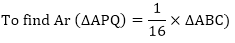In  ΔABC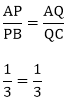According to converse of basic proportional theorem if a line divides any two sides of a triangle in the same ratio, then the line must be parallel to the third side.

Hence,

PQ ∥ BC

Hence in ΔABC and ΔAPQ

∠APQ = ∠B (corresponding angles)

∠PAQ = ∠BAC (common)

ΔABC ∼ ΔAPQ### Question: 16

If D is a point on the side AB of ΔABC such that AD: DB = 3 : 2 and E is a point on BC such that DE || AC. Find the ratio of areas of ΔABC and ΔBDE.

### Solution:

Given In ΔABC, D is appoint on the side AB such that AD: DB = 3: 2. E is a point on side BC such that DE ∥ AC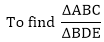In ΔABC,  ΔBDE,

∠BDE = ∠A (corresponding angles)

∠DBE = ∠ABC

ΔABC ∼ ΔBDE

We know that the ratio of the two similar triangles is equal to the ratio of the squares of their corresponding sides

Let AD = 2x and BD = 3x

Hence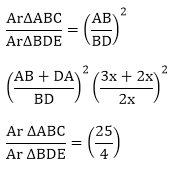### Question: 17

If ΔABC and ΔBDE are equilateral triangles, where D is the midpoint of BC, find the ratio of areas of  ΔABC and  ΔBDE.

### Solution:

Given In ΔABC, ΔBDE are equilateral triangles. D is the point of BC.In ΔABC, ΔBDE

ΔABC ∼ ΔBDE (A criteria of similarity all angles of the equilateral triangles are equal)

Since D is the mid-point of BC, BD : DC = 1

We know that the ratio of the areas of the two similar triangles is equal to the ratio of squares of their corresponding sides.

Let DC = x, and BD = x

Hence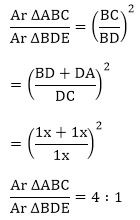### Question: 18

Two isosceles triangles have equal vertical angles and their areas are in the ratio 36: 25. Find the ratio of their corresponding heights.

### Solution:

Given:

Two isosceles triangles have equal vertical angles and their areas are in the ratio of 36: 25.

To find:

Ratio of their corresponding heights

Suppose  ΔABC and  ΔPQR are two isosceles triangles with ∠A = ∠P.

Therefore,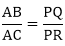In  ΔABC and ΔPQR,

∠A = ∠P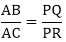∴  ΔABC ~ ΔPQR (SAS similarity)

Let AD and PS be the altitudes of ΔABC and ΔPQR, respectively.

We know that the ratio of areas of two similar triangles is equal to the ratio of squares of their corresponding altitudes.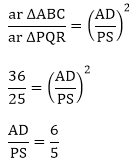Hence, the ratio of their corresponding heights is 6: 5.

### Question: 19

In the given figure. ΔABC and ΔDBC are on the same base BC. If AD and BC intersect at O, Prove that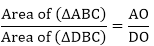### Solution:

Given ΔABC and ΔDBC are on the same BC. AD and BC intersect at O.AL⊥BC and DM⊥BC

Now, in ΔALO and ΔALO we have

∠ALO =  ∠DMO = 90°

∠AOL = ∠DOM (vertically opposite angles)

Therefore  ΔALO ∼ ΔDMO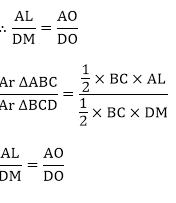### Question: 20

ABCD is a trapezium in which AB || CD. The Diagonal AC and BC intersect at O. Prove that:

(i) ΔAOB ΔCOD

(ii) If OA = 6 cm, OC = 8 cm,

Find: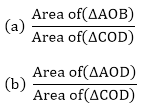### Solution:

Given ABCD is the trapezium which AB∥CD

The diagonals AC and BD intersect at o.

To prove:

(i) ΔAOB ∼ ΔCOD

(ii) If OA = 6 cm, OC = 8 cm

To find :Construction: Draw a line MN passing through O and parallel to AB and CD

Now in ΔAOB and ΔCOD

(i) Now in  ∠OAB = ∠OCD  (Alternate angles)

(ii) ∠OBA = ∠ODC       (Alternate angles)

∠AOB = ∠COD (vertically opposite angle)

ΔAOB ∼ ΔCOD (A. A. A criteria)

a) We know that the ratio of areas of two triangles is equal to the ratio of squares of their corresponding sides.b) We know that the ratio of two similar triangles is equal to the ratio of their corresponding sides.### Question: 21

In  ΔABC, P divides the side AB such that AP:PB = 1 : 2. Q is a point in AC such that PQ || BC. Find the ratio of the areas of ΔAPQ and trapezium BPQC.

### Solution:

Given: In ΔABC , P divides the side AB such that AP: PB = 1:2, Q is a point on AC on such that PQ∥BC

To find : The ratio of the areas of  ΔAPQ and the trapezium BPQC.

In ΔAPQ and ΔABC

∠APQ = ∠B (corresponding angles)

∠PAQ = ∠BAC (common)

So, ΔAPQ ∼ ΔABC (AA Similarity)

We know that the ratio of areas of the two similar triangles is equal to the ratio of the squares of their corresponding sides.Let Area of ΔAPQ = 1 sq. units and Area of ΔABC = 9x sq.units

Ar[trap BCED] = Ar(ΔABC) – Ar(ΔAPQ)

= 9x - 1x

= 8x sq units

Now,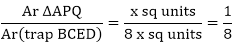## Complete JEE Main/Advanced Course and Test Series

OFFERED PRICE: Rs. 15,900
View Details Xpress Buy

### Course Features

• 731 Video Lectures
• Revision Notes
• Test paper with Video Solution
• Mind Map
• Study Planner
• NCERT Solutions
• Discussion Forum
• Previous Year Papers

### Course Features

• 728 Video Lectures
• Revision Notes
• Previous Year Papers
• Mind Map
• Study Planner
• NCERT Solutions
• Discussion Forum
• Test paper with Video Solution